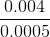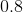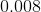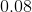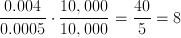# PSAT Math : Decimal Operations

## Example Questions

← Previous 1 3 4

### Example Question #1 : How To Multiply Decimals

45.728 x 3.2 = ?

14632.96

146.3296

14.63296

146329.6

1463.296

146.3296

Explanation:

Multiply the numbers out in long format, then move the decimal point over the total number of decimal points in the two numbers (3 in the first, 1 in the second, so 4 total):

45.728 * 3.2 = 91456

91456 + 1371840 = 1,463,296 → 146.3296

Or just count the decimal points in the answer and make sure it's 4.

### Example Question #2 : How To Multiply Decimals

At the farmer's market, oranges are $0.30 each, apples are$0.25 each, bananas are $0.40 each, and tomatoes are$0.60 each. If Scott buys 3 oranges, 7 apples, 4 bananas, and 8 tomatoes, how much does he spend?

$9.05$7.75

$6.80$10.40

$11.40 Correct answer:$9.05

Explanation:

To find out how much Scott spends, we need to multiply the quantity of each fruit by its price and add them all up.

3 * $0.30 + 7 *$0.25 + 4 * $0.40 + 8 *$0.60 = $9.05 ### Example Question #1 : Decimal Operations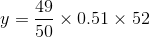Ifis 25% of, what is the closest integer to the value of? Possible Answers: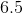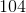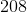Correct answer:Explanation: We can approximate the value of y pretty quickly: y ≈ 1(0.50)(52) = 26 Since y = 0.25x = (1/4)x we know that x = 4y ≈ 4(26) = 104 ### Example Question #2 : Decimal Operations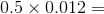Possible Answers: 6 0.006 0.06 0.6 0.0006 Correct answer: 0.006 Explanation: It's much easier to work with whole numbers, so we'll ignore the decimals for the first step: 5 x 12 = 60 Now look at the decimals. To add them back in, think of 60 as 60.0. Now move the decimal point four places to the left: 0.5 x 0.012 = .0060 = .006 ### Example Question #1 : Decimal Operations At the store, green fabric costs$4.53 a yard. How much does 3.8 yards of green fabric cost? (Round to two decimal places.)

$17.21$17.12

$17.13$17.22

$17.31 Correct answer:$17.21

Explanation:

This is a simple multiplication problem involving decimals. Simply multiply 4.53 by 3.8: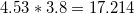Now, round to the nearest two decimal places, giving an answer of 17.21.

### Example Question #2 : Decimal Operations

Find the product. Round to the nearest hundredths place.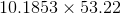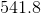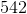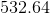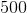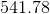Explanation:

The hundredths place is the position two places to the right of the decimal. Our product is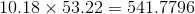. Rounding to the hundredths place results in.

### Example Question #1 : How To Divide Decimals

simplify: 0.2 ÷ 0.04

5
0.005
0.05
2
0.02
Explanation:

0.2 ÷ 0.04  <- easiest way to approach is to move the decimal to the right (the same amount of spaces on each side) so that the decimal disappears

since 0.04 has 2 spaces and 0.2 only has 1, move the decimal 2 spaces to the right on each side

0.2 ÷ 0.04 = 20 ÷ 4 = 5

### Example Question #1 : How To Divide Decimals

If the cost of 20 equally priced pencils cost $5.80, then at the same rate what is the greatest number of pencils you can purchase for$50?

200

172

150

173

172.41

172

Explanation:

Remeber for this problem that you cannot spend more than $50. First find the price of each pencil by 5.80/20 = 29 cents. And then use this to do 50/0.29 = 172.41. So the greatest number of pencils you can purchase for$50 is 172 pencils.

### Example Question #3 : How To Divide Decimals

Charlie has charges on his credit card of $63.70,$37.32, and $81.78. If he pays down half his bill at once, and then pays the rest in four equal monthly installments, how much is each monthly installment? Possible Answers: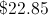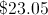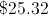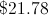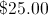Correct answer:Explanation: Adding all the charges together and dividing by 2 yields$91.40. Dividing this by 4 yields a monthly installment of \$22.85.

### Example Question #1 : Decimal Operations

Simplify: